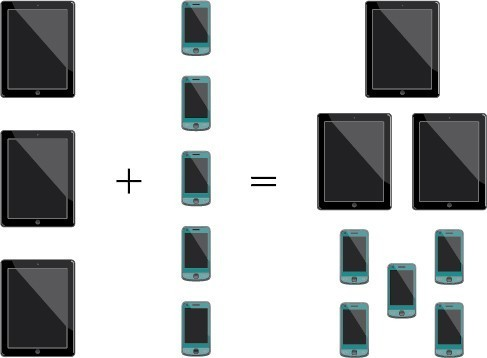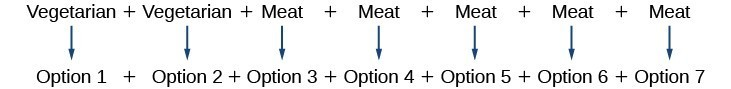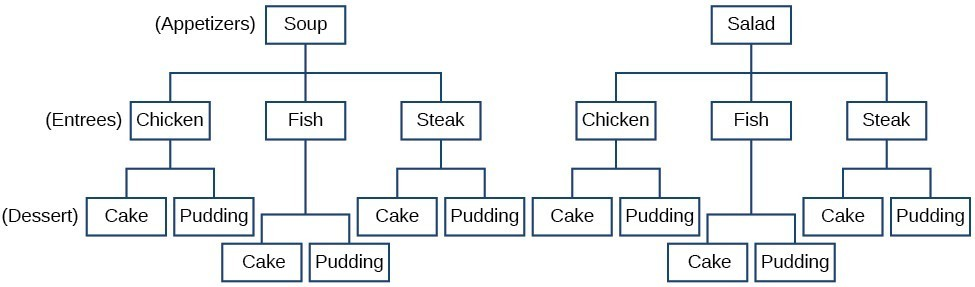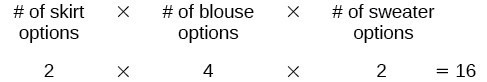## Using the Addition and Multiplication Principles

The company that sells customizable cases offers cases for tablets and smartphones. There are 3 supported tablet models and 5 supported smartphone models. The Addition Principle tells us that we can add the number of tablet options to the number of smartphone options to find the total number of options. By the Addition Principle, there are 8 total options, as we can see in Figure 1.Figure 1

### A General Note: The Addition Principle

According to the Addition Principle, if one event can occur in $m$ ways and a second event with no common outcomes can occur in $n$ ways, then the first or second event can occur in $m+n$ ways.

### Example 1: Using the Addition Principle

There are 2 vegetarian entrée options and 5 meat entrée options on a dinner menu. What is the total number of entrée options?

### Solution

We can add the number of vegetarian options to the number of meat options to find the total number of entrée options.There are 7 total options.

### Try It 1

A student is shopping for a new computer. He is deciding among 3 desktop computers and 4 laptop computers. What is the total number of computer options?

Solution

## Using the Multiplication Principle

The Multiplication Principle applies when we are making more than one selection. Suppose we are choosing an appetizer, an entrée, and a dessert. If there are 2 appetizer options, 3 entrée options, and 2 dessert options on a fixed-price dinner menu, there are a total of 12 possible choices of one each as shown in the tree diagram in Figure 2.The possible choices are:

1. soup, chicken, cake
2. soup, chicken, pudding
3. soup, fish, cake
4. soup, fish, pudding
5. soup, steak, cake
6. soup, steak, pudding

We can also find the total number of possible dinners by multiplying.

We could also conclude that there are 12 possible dinner choices simply by applying the Multiplication Principle.

### A General Note: The Multiplication Principle

According to the Multiplication Principle, if one event can occur in $m$ ways and a second event can occur in $n$ ways after the first event has occurred, then the two events can occur in $m\times n$ ways. This is also known as the Fundamental Counting Principle.

### Example 1: Using the Multiplication Principle

Diane packed 2 skirts, 4 blouses, and a sweater for her business trip. She will need to choose a skirt and a blouse for each outfit and decide whether to wear the sweater. Use the Multiplication Principle to find the total number of possible outfits.

### Solution

To find the total number of outfits, find the product of the number of skirt options, the number of blouse options, and the number of sweater options.There are 16 possible outfits.

### Try It 2

A restaurant offers a breakfast special that includes a breakfast sandwich, a side dish, and a beverage. There are 3 types of breakfast sandwiches, 4 side dish options, and 5 beverage choices. Find the total number of possible breakfast specials.

Solution